## How to Convert Square Miles to Square Meters | Area Units

The image below represents square miles to square meters.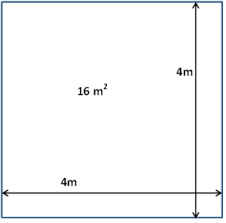To convert square miles to square meters, one essential parameter is needed and this parameter is Unit Value.

The formula for converting square miles to square meters:

1 Square Mile = 2589988 Square Meters

Let’s solve an example;
Find the conversion of square miles to square meters when the unit value is 3.

1 Square Mile = 2589988 Square Meters

Then,

3 Square Miles = (3 x 2589988) Square Meters
3 Square Miles = 7769964 Square Meters

Therefore, the square meter(s) is 7769964.

## How to Convert Acres to Square Meters | Area Units

The image below represents acres to square meters.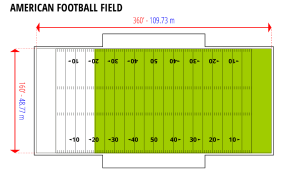To convert acres to square meters, one essential parameter is needed and this parameter is Unit Value.

The formula for converting acres to square meters:

1 Acre = 4046.856 Square Meters

Let’s solve an example;
Find the conversion of acres to square meters when the unit value is 5.

1 Acre = 4046.856 Square Meters

Then,

5 Acres = (5 x 4046.856) Square Meters
5 Acres = 20234.280000000002 Square Meters

Therefore, the square meter(s) is 20234.2

## How to Convert Square Yards to Square Meters | Area Units

The image below represents square yards to square meters.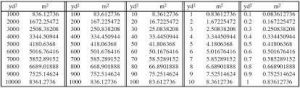To convert square yards to square meters, one essential parameter is needed and this parameter is Unit Value.

The formula for converting square yards to square meters:

1 Square Yard = 0.836127 Square Meters

Let’s solve an example;
Find the conversion of square yards to square meters when the unit value is 4.

1 Square Yard = 0.836127 Square Meters

Then,

4 Square Yards = (4 x 0.836127) Square Meters
4 Square Yards = 3.344508 Square Meters

Therefore, the square meter(s) is 3.344508.

## How to Convert Square Feet to Square Meters | Area Units

The image below represents square feet to square meters.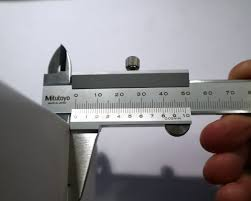To convert square feet to square meters, one essential parameter is needed and this parameter is Unit Value.

The formula for converting square feet to square meters:

1 Square Feet = 0.092903 Square Meters

Let’s solve an example;
Convert square feet to square meters when the unit value is 20.

1 Square Feet = 0.092903 Square Meters

Then,

20 Square Feet = (20 x 0.092903) Square Meters
20 Square Feet = 1.85806 Square Meters

Therefore, the square meter(s) is 1.85806.

## How to Convert Square Inches to Square Meters | Area Units

The image below represents square inches to square meters.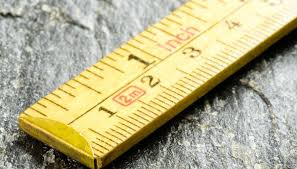To convert square inches to square meters, one essential parameter is needed and this parameter is Unit Value.

The formula for converting square inches to square meters:

1 Square Inch = 0.000645 Square Meters

Given an example;
Convert square inches to square meters when the unit value is 2.

1 Square Inch = 0.000645 Square Meters

Then,

2 Square Inches = (2 x 0.000645) Square Meters
2 Square Inches = 0.00129 Square Meters

Therefore, the square meter(s) is 0.00129.

## How to Convert Square Kilometers to Square Meters | Area Units

The image below represents square kilometers to square meters.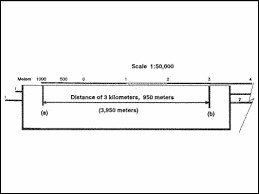The conversion of square kilometers to square meters requires one essential parameter and this parameter is Unit Value.

The formula for converting square kilometers to square meters:

1 Square Kilometer = 1000000 Square Meters

Given an example;
Convert square kilometers to square meters when the unit value is 4.

1 Square Kilometer = 1000000 Square Meters

Then,

4 Square Kilometers = (4 x 1000000) Square Meters
4 Square Kilometers = 4000000 Square Meters

Therefore, the square meter(s) is 4000000.

## How to Convert Hectares to Square Meters | Area Units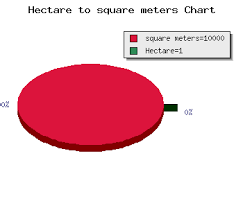The image above represents hectares to square meters.

The conversion of hectares to square meters requires one essential parameter and this parameter is Unit Value.

The formula for converting hectares to square meters:

1 Hectare = 10000 Square Meters

Given an example;
Convert hectares to square meters when the unit value is 3.

1 Hectare = 10000 Square Meters

Then,

3 Hectares = (3 x 10000) Square Meters
3 Hectares = 30000 Square Meters

Therefore, the square meter(s) is 30000.

## How to Convert Square Meters to Acres | Area UnitsThe image above represents square meters to acres.

The conversion of square meters to acres requires one essential parameter and this parameter is Unit Value.

The formula for converting square meters to acres:

1 Square Meter = 0.000247 Acres

Given an example;
Convert square meters to acres when the unit value is 4.

1 Square Meter = 0.000247 Acres

Then,

4 Square Meters = (4 x 0.000247) Acres
4 Square Meters = 0.000988 Acres

Therefore, the acre(s) is 0.000988.

## How to Convert Square Meters to Square Miles | Area Units

The image below represents square meters to square miles.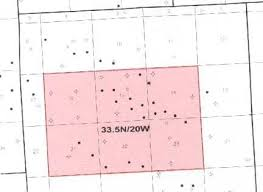The conversion of square meters to square miles requires one essential parameter and this parameter is Unit Value.

The formula for converting square meters to square miles:

1 Square Meter = 0.00000038610216 Square Miles

Given an example;
Convert square meters to square miles when the unit value is 6.

1 Square Meter = 0.00000038610216 Square Miles

Then,

6 Square Meters = (6 x 0.00000038610216) Square Miles
6 Square Meters = 0.00000231661296 Square Miles

Therefore, the square mile(s) is 0.00000231661296.

## How to Convert Square Meters to Square Yards | Area Units

The image below represents square meters to square yards.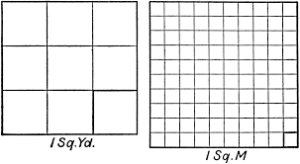The conversion of square meters to square yards requires one essential parameter and this parameter is Unit Value.

The formula for converting square meters to square yards:

1 Square Meter = 1.19599 Square Yards

Let’s solve an example;
Convert square meters to square yards when the unit value is 5.

1 Square Meter = 1.19599 Square Yards

Then,

5 Square Meters = (5 x 1.19599) Square Yards
5 Square Meters = 5.9799500000000005 Square Yards

Therefore, the square yard(s) is 5.979.